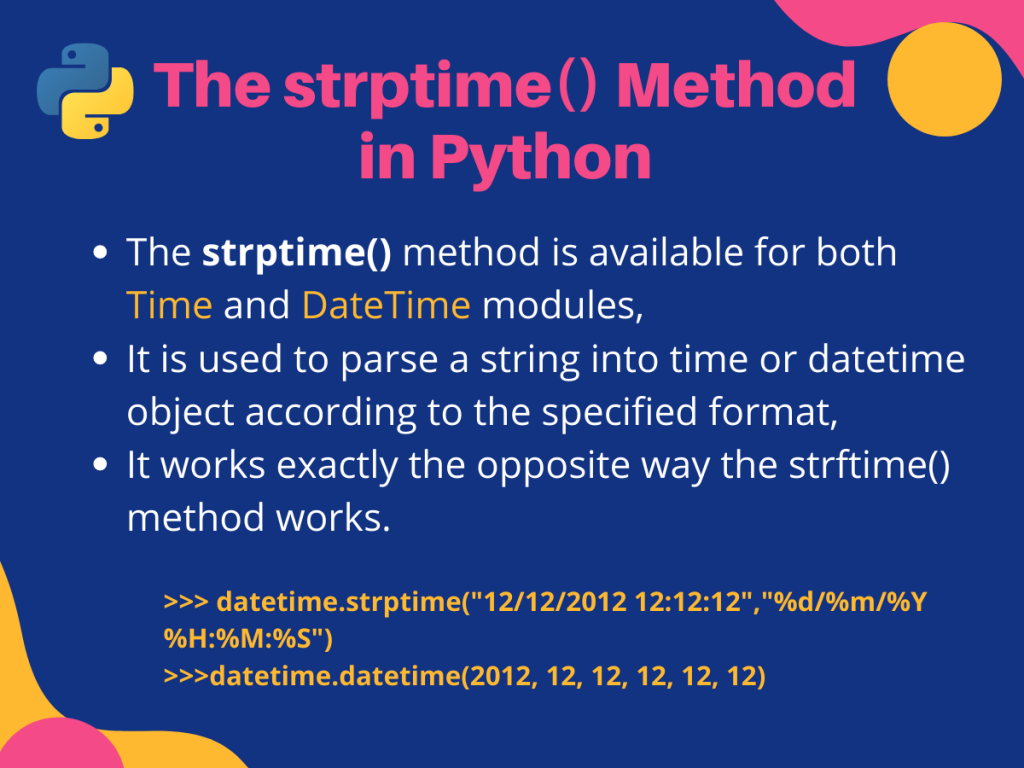# Convert string to datetime using Python strptime()So for today, we are going to discuss how we convert a string to datetime using the Python strptime() method.

Let us get started.

## Python strptime() Method

The Python `strptime()` method is available in both `datetime` and `time` modules. It is used to parse a given string into datetime or time object according to the specified format.

The use of this function is about the same for both the modules, the only difference being in the type of object returned. Let us have a closer look at the syntax for using it.

For the datetime module, the syntax for using the `strptime()` method is given below.

```datetime.strptime(date_string, format)
```

The above statement returns a `datetime` object corresponding to `date_string` parsed according to the specified format.

And for the time module, the syntax for using the `strptime()` is fairly similar.

```time.strptime(string[, format])
```

Here the method does the same job just here the returned value is a `struct_time` object as returned by `gmtime()` or `localtime()`.

For both cases, the format parameter uses the same directives as those used by strftime() as given in the format table below. The format parameter has to be a string and has a default value `"%a %b %d %H:%M:%S %Y"`. If the string cannot be parsed according to the given format, or if it has excess data after parsing, a `ValueError` is raised.

## Format Table

You must follow the below-given format table to use appropriate directives while specifying the format parameter.

## Working with the strptime() Method

So now that we are familiar with the syntax of using the `strptime()` method for both datetime and time modules, let us see how we can use it.

### 1. time.strptime()

In the example below, we are going to convert a given string into a time object.

```import time

time_string = "06/05/2020 12:06:58"

obj = time.strptime(time_string, "%d/%m/%Y %H:%M:%S")

print(type(obj))
print(obj)

#parsing using default formatting: %a %b %d %H:%M:%S %Y

def_obj = time.strptime("Wed May 06 12:06:58 2020")
print(def_obj)
```

Output:

```<class 'time.struct_time'>
time.struct_time(tm_year=2020, tm_mon=5, tm_mday=6, tm_hour=12, tm_min=6, tm_sec=58, tm_wday=2, tm_yday=127, tm_isdst=-1)
time.struct_time(tm_year=2020, tm_mon=5, tm_mday=6, tm_hour=12, tm_min=6, tm_sec=58, tm_wday=2, tm_yday=127, tm_isdst=-1)
```

Here, `time_string` is the given string containing the corresponding time parameters. We parse this string using the strptime() function following the “%d/%m/%Y %H:%M:%S” format into the variable `obj`. As expected obj is of type `time.struct_time`.

Nextly, we have also parsed another string using the default formatting(without specifying the format parameter). As mentioned earlier in this case by default format is set to `%a %b %d %H:%M:%S %Y` . This justifies the output.

### 2. datetime.strptime()

Now let us use the `strptime()` method to convert a given string into a datetime object.

```from datetime import datetime

dt_string = "06//05//2020 12:06:58"

obj = datetime.strptime(dt_string, "%d//%m//%Y %H:%M:%S")

print(type(obj))
print("DateTime object: ", obj)
print("Time object: ", obj.time())
print("Date object: ", obj.date())
```

Output:

As you can see from the output above, the `dt_string` is successfully converted into a datetime object. We can also use the `time()` and `date()` functions over this object to get datetime.time and datetime.date objects respectively.

Let us look at another example where we take the date and time using the input() function from the user.

```from datetime import datetime

dt_string = str(input("Enter date in dd:mm:yyyy format:"))

try:
obj = datetime.strptime(dt_string, "%d:%m:%Y")
print(obj)
print(obj.strftime("Date:%d Month:%m Year:%Y")) #strftime to format datetime obj
except ValueError as ex:
print("ValueError: ", ex)
```

Output:

In this example, we take the `dt_string` as user input and convert it into a datetime object using the `strptime()` method. Again we take this object and print it is our desired format using the `strftime()` method.

What if we do not provide the strptime() method a string which matches to the specified format? In that case, a `ValueError` is raised. Look at the output below(for the same code) where we do so.

```Enter date in dd:mm:yyyy format:6/5/20
ValueError:  time data '6/5/20' does not match format '%d:%m:%Y'
```

## Summing Up

So we today learned about converting a string into various types in Python using the `strptime()` method.

For any further questions related to the topic feel free to use the comments below.